# Bernoulli’s Principle

Bernoulli’s principle formulated by Daniel Bernoulli states that as the speed of a moving fluid increases (liquid or gas), the pressure within the fluid decreases. Although Bernoulli deduced the law, it was Leonhard Euler who derived Bernoulli’s equation in its usual form in the year 1752.

## What is Bernoulli’s Principle?

Bernoulli’s principle states that

The total mechanical energy of the moving fluid comprising the gravitational potential energy of elevation, the energy associated with the fluid pressure and the kinetic energy of the fluid motion, remains constant.

Bernoulli’s principle can be derived from the principle of conservation of energy.

## Bernoulli’s Principle Formula

Bernoulli’s equation formula is a relation between pressure, kinetic energy, and gravitational potential energy of a fluid in a container.

The formula for Bernoulli’s principle is given as follows:

$$\begin{array}{l}p + \frac{1}{2} \rho v^2 + \rho gh =constant\end{array}$$

Where p is the pressure exerted by the fluid, v is the velocity of the fluid, ρ is the density of the fluid and h is the height of the container.

Bernoulli’s equation gives great insight into the balance between pressure, velocity and elevation.

Related Articles:

## Bernoulli’s Equation Derivation

Consider a pipe with varying diameter and height through which an incompressible fluid is flowing. The relationship between the areas of cross-sections A, the flow speed v, height from the ground y, and pressure p at two different points 1 and 2 are given in the figure below.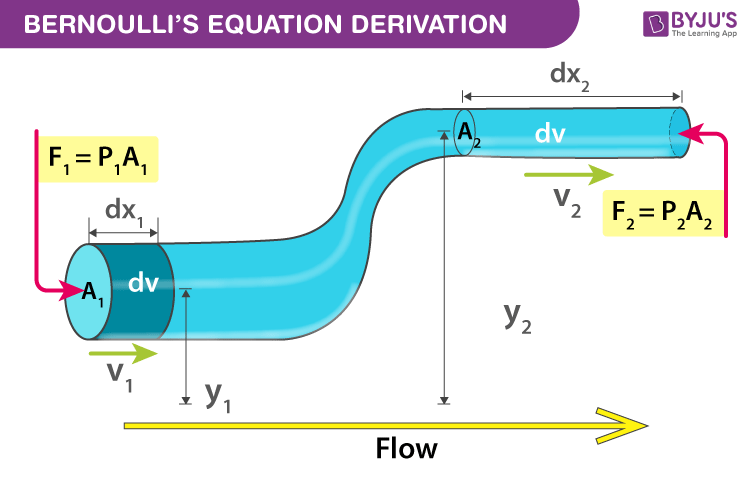Bernoulli’s Principle & Equation !
Assumptions:

• The density of the incompressible fluid remains constant at both points.
• The energy of the fluid is conserved as there are no viscous forces in the fluid.

Therefore, the work done on the fluid is given as:

dW = F1dx1 – F2dx2

dW = p1A1dx1 – p2A2dx2

dW = p1dv – p2dv = (p1 – p2)dv

We know that the work done on the fluid was due to the conservation of change in gravitational potential energy and change in kinetic energy. The change in kinetic energy of the fluid is given as:

$$\begin{array}{l}dK = \frac{1}{2}m_{2}v_{2}^{2}-\frac{1}{2}m_{1}v_{1}^{2}=\frac{1}{2}\rho dv(v_{2}^{2}-v_{1}^{2})\end{array}$$

The change in potential energy is given as:

dU = m2gy2 – m1gy1 = ρdvg(y2 – y1)

Therefore, the energy equation is given as:

dW = dK + dU

$$\begin{array}{l}(p_1-p_2)dv=\frac{1}{2} \rho dv(v_{2}^{2}-v_{1}^{2})+ \rho dvg(y_{2}-y_{1})\end{array}$$

$$\begin{array}{l}p_1-p_2)=\frac{1}{2} \rho (v_{2}^{2}-v_{1}^{2})+ \rho g(y_{2}-y_{1})\end{array}$$

Rearranging the above equation, we get

$$\begin{array}{l}p_{1}+\frac{1}{2}\rho v_{1}^{2}+\rho gy_{1}=p_{2}+\frac{1}{2}\rho v_{2}^{2}+\rho gy_{2}\end{array}$$

This is Bernoulli’s equation.## Principle of Continuity

According to the principle of continuity

If the fluid is in streamline flow and is in-compressible then we can say that mass of fluid passing through different cross sections are equal.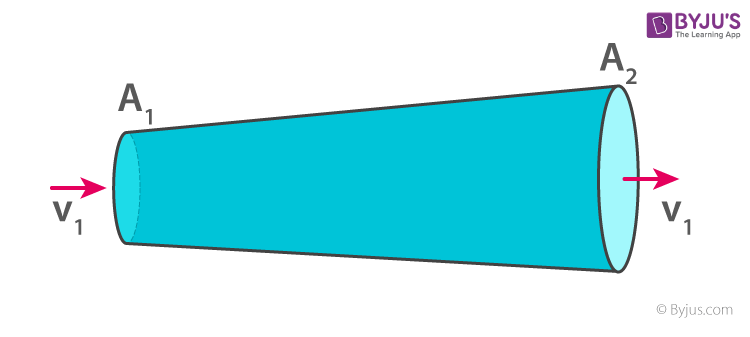From the above situation, we can say the mass of liquid inside the container remains the same.

The rate of mass entering = Rate of mass leaving

The rate of mass entering = ρA1V1Δt—– (1)

The rate of mass entering = ρA2V2Δt—– (2)

Using the above equations,

ρA1V1=ρA2V2

This equation is known as the Principle of Continuity.
Suppose we need to calculate the speed of efflux for the following setup.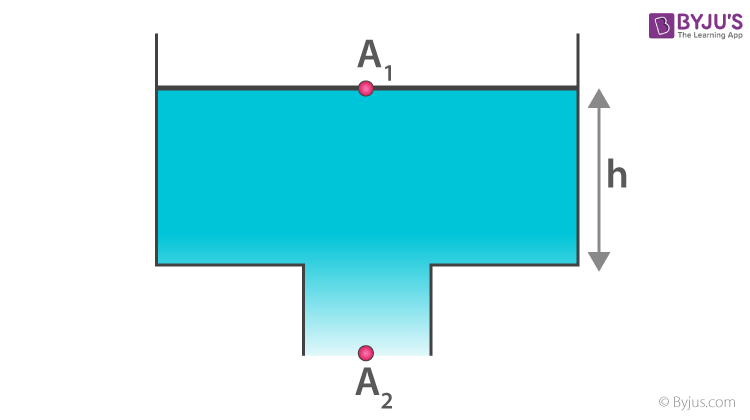Using Bernoulli’s equation at point 1 and point 2,

$$\begin{array}{l}p+\frac{1}{2}\rho v_{1}^{2}+\rho gh=p_{0}+\frac{1}{2}\rho v_{2}^{2}\end{array}$$

$$\begin{array}{l}v_2^2 = v_1^2 + 2p\rho – 2p_0\rho + 2gh\end{array}$$

Generally, A2 is much smaller than A1; in this case, v12 is very much smaller than v22 and can be neglected. We then find,
$$\begin{array}{l}v_{2}^{2}=2\frac{p-p_{0}}{\rho }+2gh\end{array}$$

Assuming A2<<A1,

We get,

$$\begin{array}{l}v_2=\sqrt{2gh}\end{array}$$

Hence, the velocity of efflux is

$$\begin{array}{l}\sqrt{2gh}\end{array}$$

## Applications of Bernoulli’s Principle and Equation

Bernoulli’s principle is used for studying the unsteady potential flow which is used in the theory of ocean surface waves and acoustics. It is also used for approximation of parameters like pressure and speed of the fluid.

The other applications of Bernoulli’s principle are:

• Venturi meter: It is a device that is based on Bernoulli’s theorem and is used for measuring the rate of flow of liquid through the pipes. Using Bernoulli’s theorem, Venturi meter formula is given as:
 $$\begin{array}{l}V=A_{1}A_{2}\sqrt{\frac{2hg}{A_{1}^{2}-A_{2}^{2}}}\end{array}$$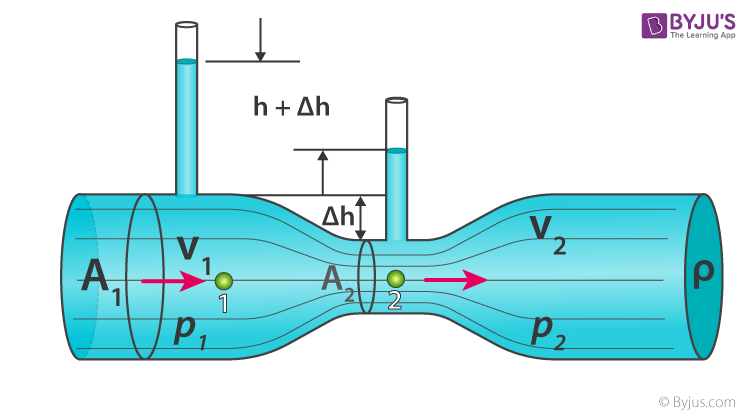• Working of an aeroplane: The shape of the wings is such that the air passes at a higher speed over the upper surface than the lower surface. The difference in airspeed is calculated using Bernoulli’s principle to create a pressure difference.
• When we are standing at a railway station and a train comes we tend to fall towards the train. This can be explained using Bernoulli’s principle as the train goes past, the velocity of air between the train and us increases. Hence, from the equation, we can say that the pressure decreases. So the pressure from behind pushes us towards the train. This is based on Bernoulli’s effect.### Relation between Conservation of Energy and Bernoulli’s Equation

Conservation of energy is applied to the fluid flow to produce Bernoulli’s equation. The net work done results from a change in a fluid’s kinetic energy and gravitational potential energy. Bernoulli’s equation can be modified depending on the form of energy involved. Other forms of energy include the dissipation of thermal energy due to fluid viscosity.

### Bernoulli’s Equation at Constant Depth

When the fluid moves at a constant depth that is when h1 = h2, then Bernoulli’s equation is given as:

$$\begin{array}{l}P_{1}+\frac{1}{2}\rho v_{1}^{2}=P_{2}+\frac{1}{2}\rho v_{2}^{2}\end{array}$$

### Bernoulli’s Equation for Static Fluids

When the fluid is static, then v1 = v2 = 0, then Bernoulli’s equation is given as:

 When v1 = v2 = 0 P1 + ρgh1 = P2 + ρgh2 When h2 = 0 P2 = P1 + ρgh1

## Bernoulli’s Principle Example

Q1. Calculate the pressure in the hose whose absolute pressure is 1.01 x 105 N.m-2 if the speed of the water in the hose increases from 1.96 m.s-1 to 25.5 m.s-1. Assume that the flow is frictionless and density 103 kg.m-3

Ans: Given,

Pressure at point 2, p2 = 1.01 × 105 N.m-2

Density of the fluid, ρ = 103 kg.m-3

Velocity of the fluid at point 1, v1 = 1.96 m.s-1

Velocity of the fluid at point 2, v2 = 25.5 m.s-1

From Bernoulli’s principle for p1,

$$\begin{array}{l}p_{1}=p_{2}+\frac{1}{2}\rho v_{2}^{2}-\frac{1}{2}\rho v_{1}^{2}=p_{2}+\frac{1}{2}\rho (v_{2}^{2}-v_{1}^{2})\end{array}$$

Substituting the values in the above equation, we get

$$\begin{array}{l}p_{1}= (1.01\times 10^{5})+\frac{1}{2}(10^{3})[(25.5)^{2}-(1.96)^{2}]\end{array}$$

p1 = 4.24 × 105 N.m-2

## Curve of a Baseball

Have you ever seen a baseball pitcher deliver a curveball? When the baseball takes a curved trajectory as it passes the plate, in most cases, the batter would not be able to judge the path of the ball and miss the ball. One of the crucial aspects of curveball can be explained using a formula typically used to describe fluid flow. Bernoull’s equation can be used to explain the basic aspect of the curve of a baseball (curveball). Usually, Bernoulli’sBernoulli’s equation points to pressure, height, and velocity (“air” is the fluid). At any particular point in the fluid (air), the K (constant) will be equal to the total sum of the other three values (height, pressure, and velocity).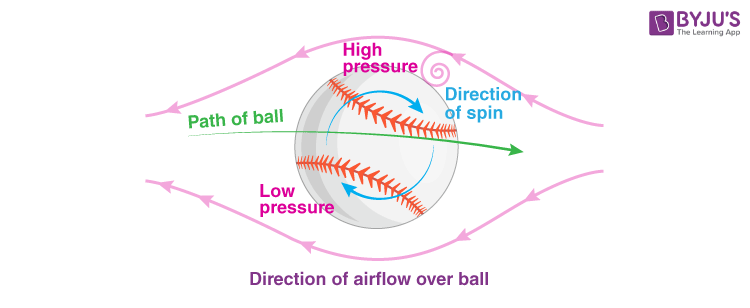## Airfoil and Bernoulli’s Principle

The air over the top of a typical airfoil encounter compressed flow lines and boosted air speed compared to the wing. This introduces a reduction in pressure on the top (as per the Bernoulli equation) and produces a lift force. Aerodynamicists use the Benaoulli concept to explain the pressure calculations made in wind tunnels. They show that when pressure calculations are done at multiple places around the airfoil and added together, it is similar to the observed lift.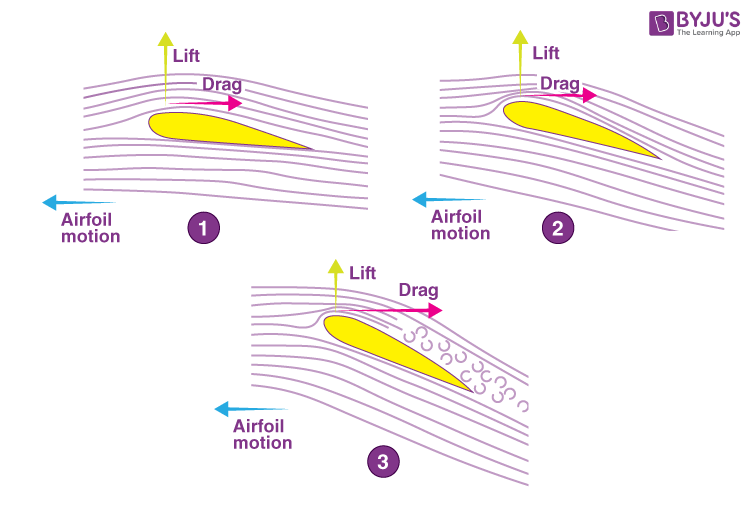## Frequently Asked Questions – FAQs

Q1

### What is Bernoulli famous for?

Daniel Bernoulli explained how the speed of fluid affects the pressure of the fluid, which is known as Bernoulli’s effect and explained the kinetic theory of gases. These two were his greatest contributions to Science, and the two concepts made him famous.

According to Bernoulli’s effect, he tried to explain that when a fluid flows through a region where the speed increases, the pressure will decrease. Bernoulli’s effects find many real-life applications, such as aeroplane wings are used for providing a lift to the plane.

Q2

### What does Bernoulli’s equation mean?

The Bernoulli equation is considered the statement of the energy conservation for the fluids that flow. This is considered to be the qualitative behaviour that lowers the pressure in the regions with high velocities. This is termed the Bernoulli effect.

Q3

### What is head loss in Bernoulli’s equation?

The head loss in Bernoulli’s equation represents the reduction in the total pressure, which is the sum of the velocity head, pressure head, and the elevation head of the fluid flowing through the hydraulic system.

Q4

### What is head loss equation?

The following equation is the mathematical representation of the head loss:
hL = f L/D × v2/2g
hL = fLv2 / 2Dg
Where,

• hL is the head loss
• f is the Darcy friction factor
• L is the pipe length
• D is inside pipe diameter
• v is the fluid velocity
• g is the gravitational constant
Q5

### What is the maximum suction head of a pump?

The maximum suction head of a pump is approximately 15 feet.

Hope you have understood the Bernoulli equation and its derivation and applications. To know more about various interesting science and maths topics, visit BYJU’S – The Learning App!.

Test your knowledge on Bernoulli's principle